Computer Science

# Lab 4 - While

## What Does It Draw?

For each exercise, draw out what you think the resulting Bit world would be.

Then paste the code into script and run it, stepping through each step.

Were there any surprises? Were there any interesting ideas?

Discuss with a partner. Click the Results link to see the answer.

### start-from-empty

from byubit import Bit

@Bit.run_from_empty(5, 3)
def go(bit):
while bit.front_clear():
bit.move()
bit.paint('green')

bit.left()

while bit.front_clear():
bit.paint('blue')
bit.move()
Result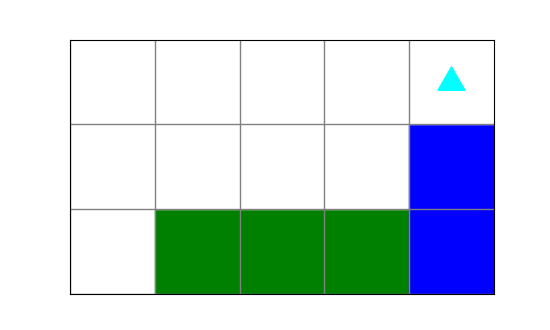### find-a-way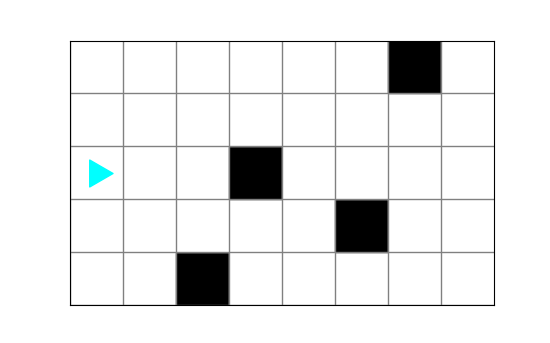from byubit import Bit

@Bit.run('find-a-way')
def go(bit):
while bit.front_clear():
bit.move()

bit.left()

while bit.front_clear():
bit.move()

bit.right()

while bit.front_clear():
bit.move()

bit.right()

while bit.front_clear():
bit.move()
bit.paint('green')
Result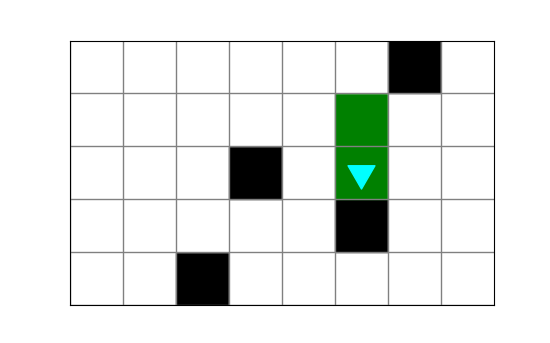### red-spot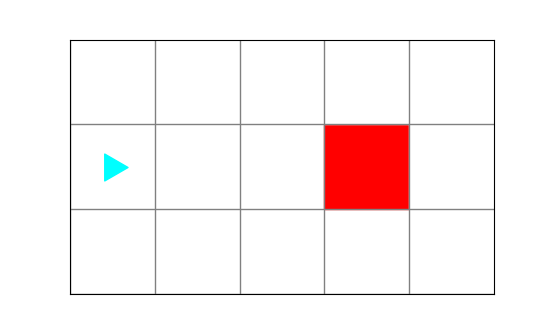from byubit import Bit

@Bit.run('red-spot')
def go(bit):
while not bit.is_red():
bit.move()

bit.left()
bit.move()
bit.left()

while bit.front_clear():
bit.move()
bit.paint('blue')

Result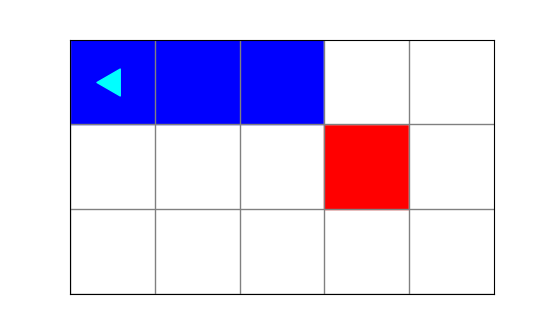## Copy Cat

Using the provided code stub, write code to produce the following images.

from byubit import Bit

@Bit.run_from_empty(5, 3)
def go(bit):
pass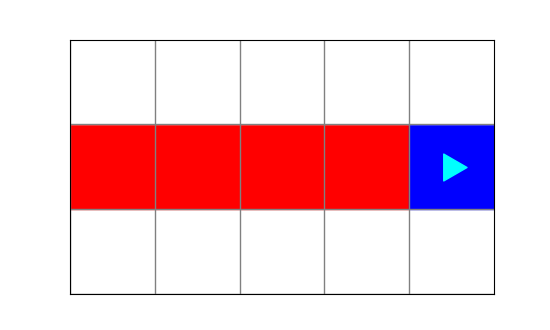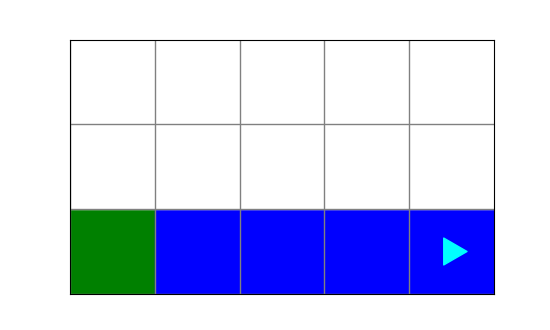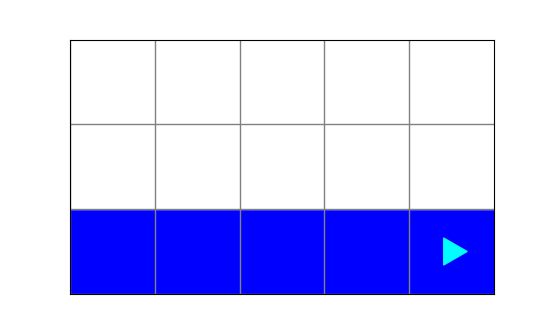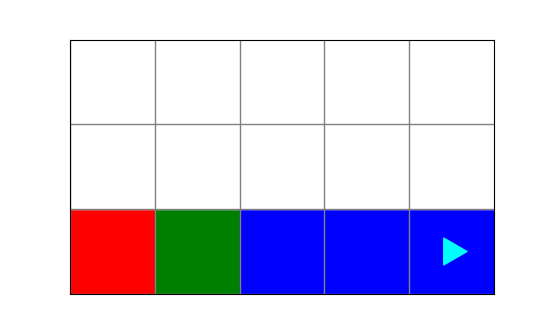## Activities

For each of the following puzzles:

1. Draw out a strategy for solving the puzzle
2. What are the subgoals of the problem?
3. What glue code is needed inbetween each goal?
4. Are there any interesting boundary conditions?
5. Implement the function in the associated file with your solution

### dive_for_treasure.py

Bit is diving for treasure!

First get Bit to the ocean, then dive to the red treasure and take it (leaving blue ocean behind).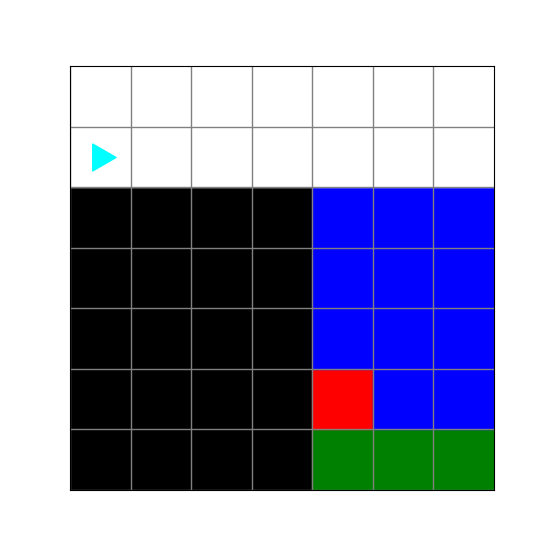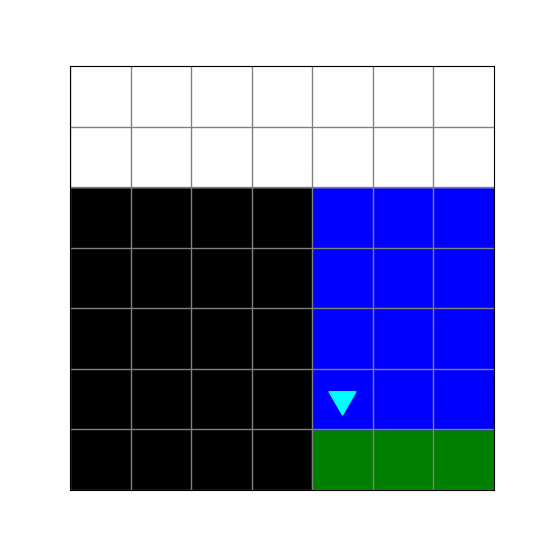### go_to_lake.py

Bit wants to get to the lake. He doesn’t know how to get there. He asks for directions.

Bit: “How do I get to the lake?”

Helpful passerby: “Take a left at the stop sign.”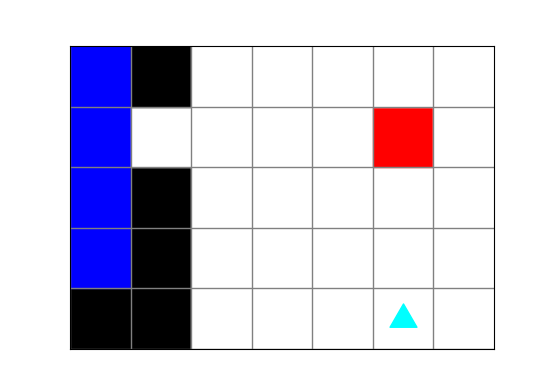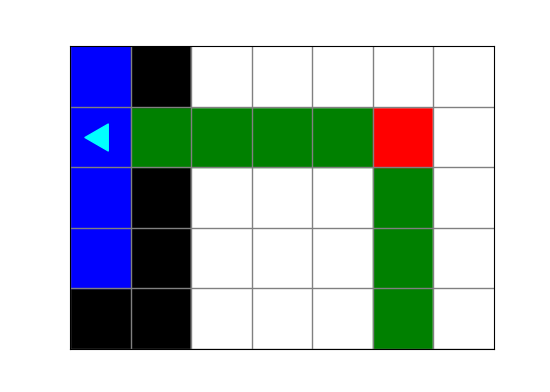### nice_blue_square.py

Bit is trying to paint a square…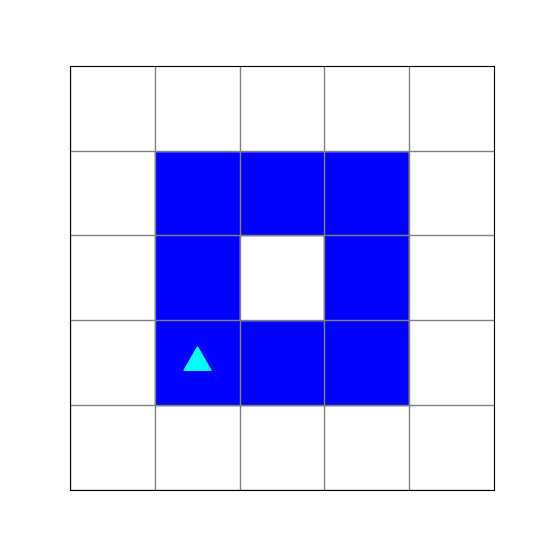…but something isn’t working right.

### roof_the_house.py

Help Bit build a roof for his house.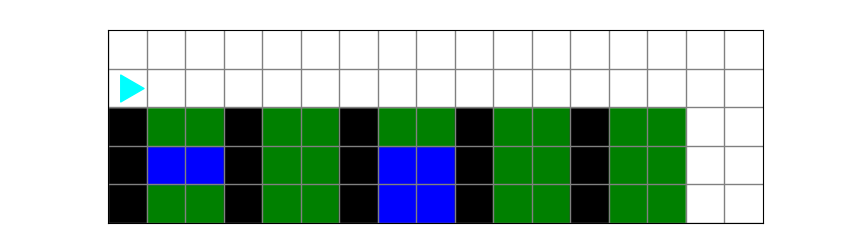The black columns represent supports.

The roof can extend 3 squares beyond a support. If there is another support under the third square, the roof can continue to extend.

In other words:

• If Bit is over a black square, Bit can move three more squares before checking again for a black square.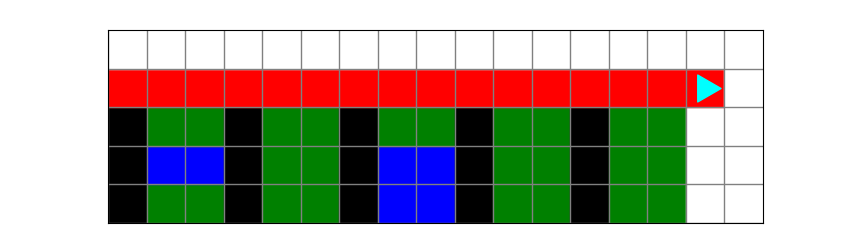### green_bucket.py

The blue handles are already in place, but the bucket is missing!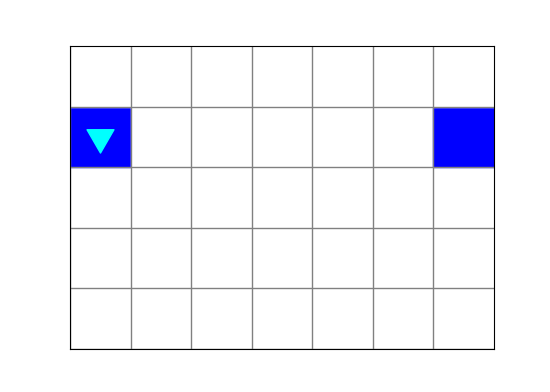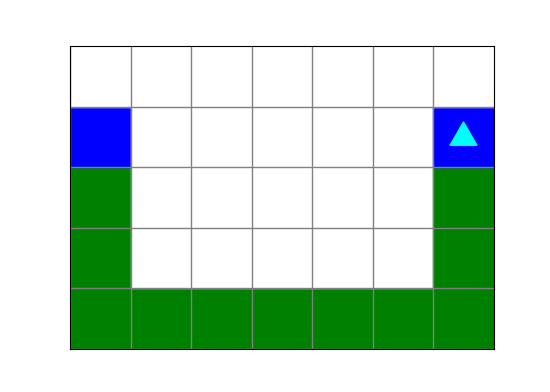### red_line.py

Bit needs to leave his room (black squares), finish the red line, and return to his room.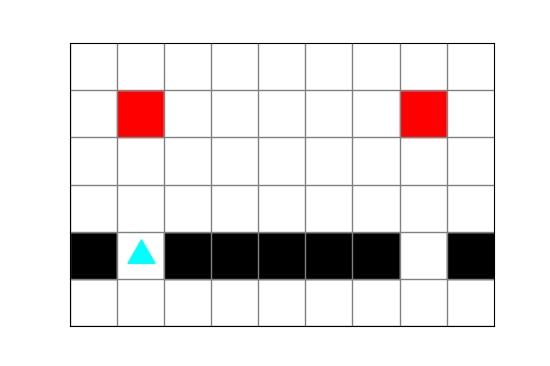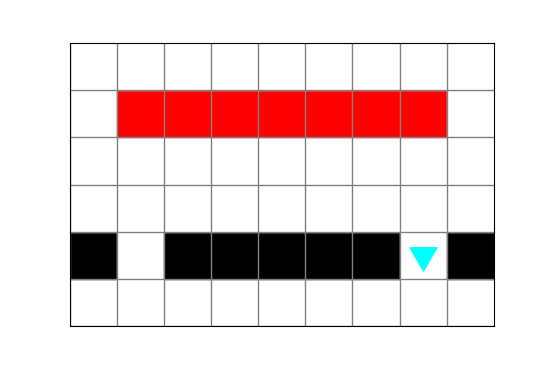dive_for_treasure.py5
go_to_lake.py5
nice_blue_square.py5
roof_the_house.py5
green_bucket.py5
red_line.py5